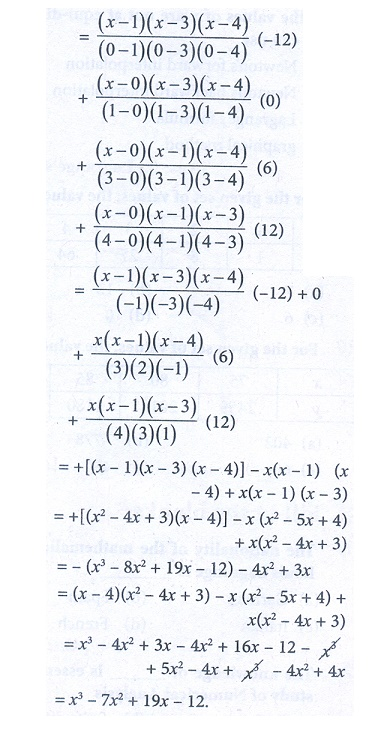Home | | Business Maths 12th Std | Miscellaneous Problems

# Miscellaneous Problems

Book back answers and solution for Exercise questions - Maths: Numerical Methods: Miscellaneous Problems

Miscellaneous Problems

1. If f ( x) = eax then show that f ( 0), ╬ö f ( 0), ╬ö2 f ( 0) are in G.P2. Prove that i) ( 1 + ╬ö )( 1 - Ōłć) = 1 ii) ╬ö Ōłć = ╬ö ŌłÆ Ōłć (iii) E Ōłć = ╬ö  = ŌłćE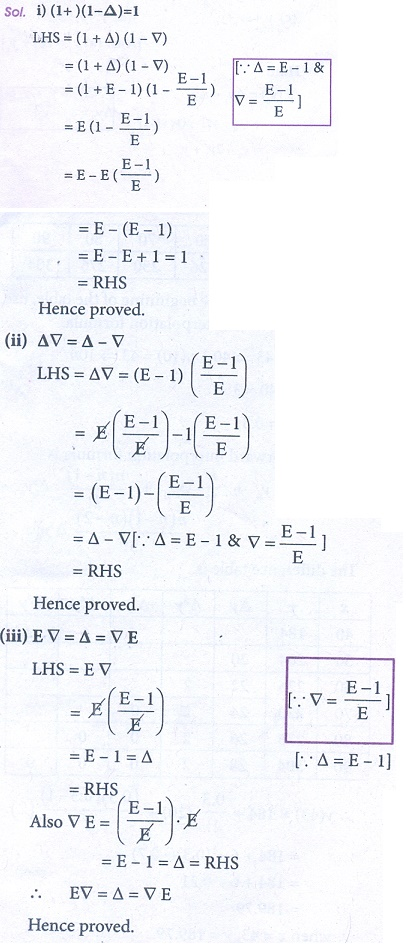3. A second degree polynomial passes though the point (1,-1) (2,-1) (3,1) (4,5). Find the polynomial.4. Find the missing figures in the following table5. Find f ( 0.5) if f ( ŌłÆ1) = 202, f ( 0) = 175 , f (1) = 82 and f ( 2) = 556. From the following data find y at x = 43 and x = 847. The area A of circle of diameter ŌĆśdŌĆÖ is given for the following values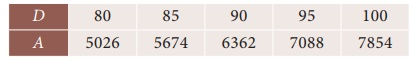Find the approximate values for the areas of circles of diameter 82 and 91 respectively.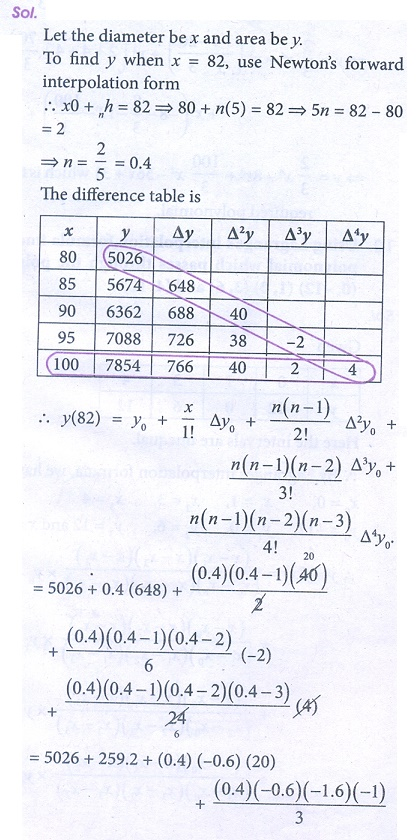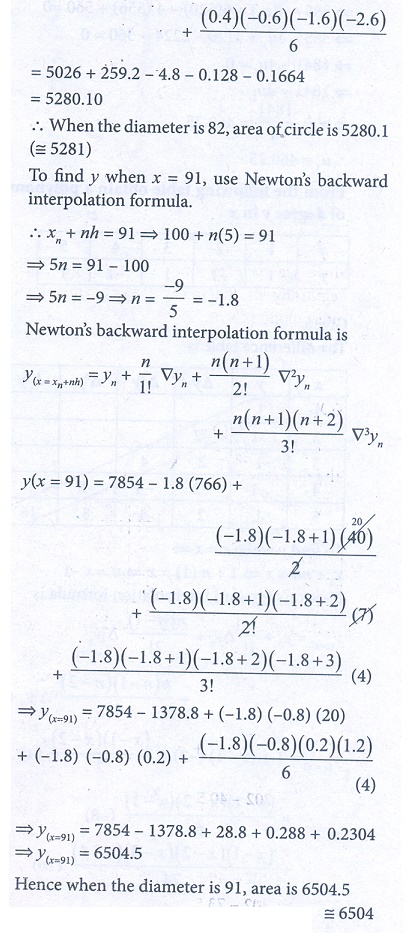8. If u0 = 560, u1 = 556, u2 = 520, u4 = 385, show that u3 = 4659. From the following table obtain a polynomial of degree y in x10. Using LagrangeŌĆÖs interpolation formula find a polynomial which passes through the points (0, ŌĆō12), (1, 0), (3, 6) and (4,12).